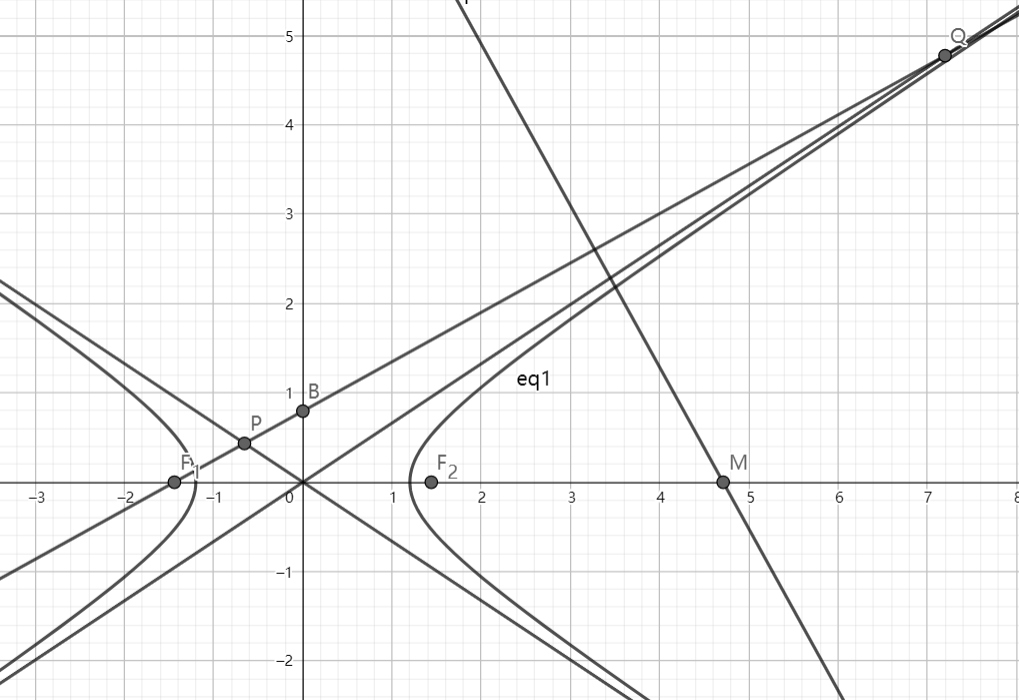# SAT1000 - P760

Geometry Level pendingAs shown above, $F_1, F_2$ are left and right focus of the hyperbola: $\dfrac{x^2}{a^2}-\dfrac{y^2}{b^2}=1\ (a,b>0)$ respectively, and $B(0,b)$.

Line $F_1B$ intersects with the two asymptotes of the hyperbola at $P,Q$, and the perpendicular bisector of $PQ$ intersects with x-axis at point $M$.

If $|MF_2|=|F_1F_2|$, then find the eccentricity of the hyperbola.

Let $E$ denote the eccentricity, submit $\lfloor 1000E \rfloor$.

Have a look at my problem set: SAT 1000 problems

×